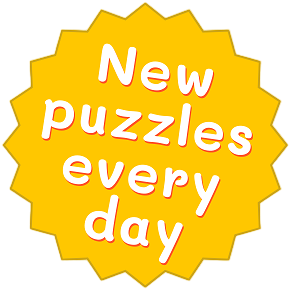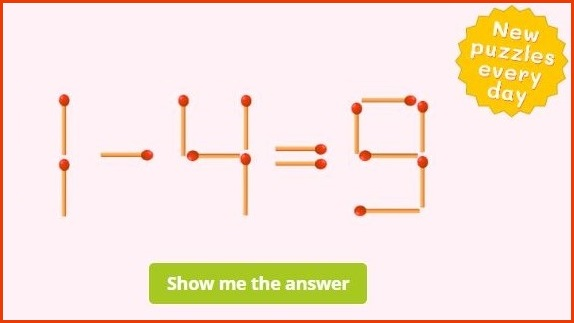﻿ Math Matchstick Puzzles with Answers PDF + Online

Page No. 1054

# Matchstick puzzle for TODAY

Math matchstick puzzles games. Every day a new matchstick game online. Move one match to fix the equation. matches puzzles. Move match stick puzzles games online. Download math matchstick puzzles with answers in PDF. Matches riddle. Easy matchstick puzzles online. Online game with matchsticks. Matches game puzzle with solution. brain teasers matchstick puzzles with answers. match stick math puzzle of the day. matches riddles. Math puzzles with matchsticks logic game. Move one matchstick riddle. Move one stick puzzle. Online puzzles with matches. Matchstick problems. Easy matchstick math puzzles with answers PDF. Match equations. Math matchstick brain teasers. Matchstick number puzzles. matchstick puzzles solutions PDF. Matchstick equation puzzles with answers. Learn addition using matchsticks. math puzzles with matchsticks. matchstick puzzles numbers.###### The possible solution:Move 1 stick to fix the equation.    📄   PRINT IT

## Math matchstick puzzles gamesMove only one matchstick so that the equation becomes correct. Various solutions for a matchstick puzzle are also possible. Click the green button to see one of the correct answers. Come back tomorrow for a new matchstick riddle!

## Matchstick brain teasers

100 Matchstick Puzzles in PDF

Printable math matchstick puzzles with answers. Move 1 stick to fix the equation.

US\$ 7.00

GET IT NOW

## Free matchstick puzzles with answers in PDF

A new matchstick game is created every day! You can print it out or download matchstick puzzles with answers in PDF. These matchstick math puzzles are a great way to challenge your brain!

These matches riddles are great as warm-up and/or cool-down activities at the beginning of math lesson, as math exercises during school holidays or as math brain teasers for all ages. Check out all our printable math games here.

## Matchstick equation puzzles with answers

Math matchstick puzzles are a type of math puzzles that involves rearranging matchsticks to fix mathematical equations. The matchsticks are used to represent numbers, symbols, or mathematical operations.

### Math puzzles with matchsticks

Here's an example of a matchstick math puzzle. Consider the equation: 1 - 4 = 9. Now, your task is to move one matchstick to fix the equation.To solve the puzzle, you can move one matchstick from the number 9 (to create number 5) and change the minus sign to an addition sign.

The modified equation becomes: 1 + 4 = 5, which is now correct.

## Matchstick math puzzles with answers PDF

These matches puzzles require creative thinking and problem-solving skills to manipulate the matchsticks and find a solution. By moving or removing matchsticks, you can change the equation to make it mathematically correct or to solve a given math problem.

Our math matchstick puzzles range from simple arithmetic operations such as addition and subtraction to more complex equations involving multiplication and division problems.

To teach simple addition and subtraction equations you can also use these online math crosswords, for improving multiplication and divison use these multiplication crosswords.

## Matchstick game ONLINE

You can download daily matchstick puzzle with a solution in PDF or you can play our matchstick game online on computer, smartphone or tablet. Matchstick games are a popular pastime for puzzle enthusiasts and can be enjoyed both individually and in a social setting, encouraging collaboration and friendly competition.

### Game with matchsticks

Math matchstick puzzles vary in difficulty and can be a fun way to challenge yourself or others while exploring mathematical concepts. See some tricks how to solve matchstick puzzles here.

## Benefits of matchstick number puzzles

Matchstick math puzzles offer a range of cognitive and educational benefits while providing an enjoyable and challenging activity for kids and adults:

1. Problem-solving skills: Matchstick math puzzles challenge your problem solving skills because they require you to think creatively and strategically. Matchstick number puzzles involve mathematical concepts and logic and encourage you to analyse and find solutions.
2. Mental agility: When you are trying to solve math matchstick puzzles you are improving your mental agility. This matchstick logic game demand quick thinking and the ability to visualize and manipulate numbers.
3. Spatial reasoning: Our math puzzles with matchsticks involve arranging matchsticks to fix the equation. This promotes spatial reasoning skills and helps improve your ability to visualize and mentally manipulate objects in space.
4. Mathematical understanding: Matchstick equation puzzles involve mathematical operations, such as addition, subtraction, multiplication, and division. By solving these match stick equations, you strengthen your mathematical skills and gain a better understanding of numerical relationships. You can use real matches or other sticks to play in classroom. This makes it easier for the children to visualize the example. In addition, by involving the fine motor skills of the hands, kids connect both brain hemispheres which leads to calming down and improving of learning.
5. Patience and perseverance: Some matchstick math problems can be quite challenging and require patience and perseverance to solve. Engaging with these matches games can help develop your determination, persistence, and willingness to tackle difficult problems.
6. Enjoyment and entertainment for all ages: Matchstick riddles are a fun and engaging form of entertainment. Move one stick puzzles provide a break from routine activities and offer a mentally stimulating way to pass the time, whether you're solving them alone or in a group.
7. Educational tool: Brain teasers with matchsticks can be used as an educational tool to introduce and reinforce mathematical concepts in a playful and interactive manner. Matchstick puzzle games can be incorporated into math lessons or used as brain teasers to promote mathematical thinking and problem-solving skills in students.

## Matchstick puzzles online

Incorporating our simple and fun online matchstick riddles and picture math puzzles can easily help your kids strengthen math and logical skills. And they won't even realize how much this is growing their brain because they'll have fun doing all these matchstick math problems!

You have no longer to print out these matchstick puzzles, you can play them online. But of course, if you want to reduce the screen time you can download printable matchstick puzzles in PDF too.

## Matchstick math game

Solving match stick puzzles strengthens logical thinking skills and develop logical reasoning skills. Logical reasoning will teach kids to think critically and solve problems creatively.

This topic seems to be sorely lacking in typical math curriculum options. In standard textbooks you may see the occasional "critical thinking" problem thrown in, but even these are usually not very good and not very engaging for kids. Online matchstick math game is loved by kids.

## Matchstick riddle

Our matchstick riddles contain easy matchstick puzzles and also some more difficult matchstick math puzzles. So it is possible both beginners and experienced puzzlers could get something out of solving math matchstick puzzles.

These matchstick math games often have multiple solutions, allowing for creative problem-solving approaches. They encourage individuals to think outside the box and explore alternative strategies. You can experiment with different arrangements of matches to find a solution.

## Brain teasers matchstick puzzles ONLINE

Working through challenging brain teasers with matchstick requires patience and concentration as the logic is teased out and the final solution verified. Practice with these types of matchstick puzzles helps the brain make connections and get used to focused concentration on a single problem.

## Matchstick math problems

Matchstick math puzzles are a type of logic puzzle that uses matchsticks to represent numbers and mathematical equations.

The task is to move one matchstick to make the equation correct. Some matchstick number puzzles have multiple solutions, while others have only 1 answer.

### Matchstick brain teasers

To solve these matchstick brain teasers, you need to carefully examine the equation and figure out which matchstick you need to move to make the equation correct. You also need to be careful not to move any matchsticks that are not necessary, as this will invalidate the solution.

Once you have solved a matchstick math problems, you can try to find other solutions. There may be multiple solutions to some matchstick brain teasers and it can be fun to see how many different solutions you can find.

Category: